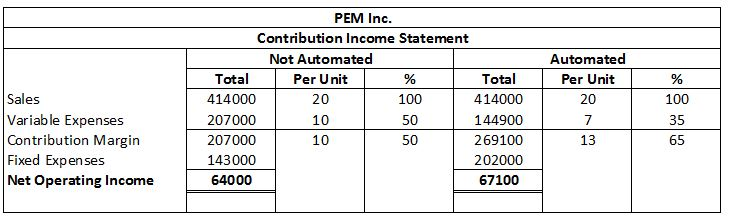# Question & Answer: Due to erratic sales of its sole product—a high-capacity battery for laptop computers—PEM, Inc., has been experiencing…..

Due to erratic sales of its sole product—a high-capacity battery for laptop computers—PEM, Inc., has been experiencing financial difficulty for some time. The company’s contribution format income statement for the most recent month is given below:

Don't use plagiarized sources. Get Your Custom Essay on
Question & Answer: Due to erratic sales of its sole product—a high-capacity battery for laptop computers—PEM, Inc., has been experiencing…..
GET AN ESSAY WRITTEN FOR YOU FROM AS LOW AS \$13/PAGE
 Sales (12,800 units × \$20 per unit) \$ 256,000 Variable expenses 128,000 Contribution margin 128,000 Fixed expenses 143,000 Net operating loss \$ (15,000 )

Required:

5. Refer to the original data. By automating, the company could reduce variable expenses by \$3 per unit. However, fixed expenses would increase by \$59,000 each month.

a. Compute the new CM ratio and the new break-even point in unit sales and dollar sales.

b. Assume that the company expects to sell 20,700 units next month. Prepare two contribution format income statements, one assuming that operations are not automated and one assuming that they are. (Show data on a per unit and percentage basis, as well as in total, for each alternative.)

c. Would you recommend that the company automate its operations?

Contribution Margin ratio = Contribution Margin / Sales * 100
Contribution Margin per unit = Selling Price per unit – Variable Cost per unit
Selling Price per unit = \$20
Current Variable Cost per unit = 128,000 / 12,800 = \$10

Expected Variable Cost per unit = \$10 – \$3 = \$7
Expected Contribution Margin per unit = \$20 – \$7 = \$13
New Contribution Margin ratio = 13 / 20 * 100
New Contribution Margin ratio = 65%

Break Even Point (in Units) = Fixed Cost / Contribution Margin per unit
Revised Fixed Expense = \$143,000 + \$59,000
Revised Fixed Expense = \$202,000

Break Even Point (in Units) = 202,000 / 13
Break Even Point (in Units) = 15,538.46 or 15,538 units

Break Even Point (in Dollar) = Fixed Cost / Contribution margin Ratio
Break Even Point (in Dollar) = 202,000 / 0.65
Break Even Point (in Dollar) = \$310,769.23